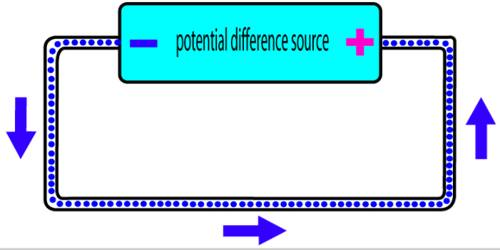Physics

# Potential DifferencePotential difference

Definition: The difference of potentials at two points in an electric field is called potential difference. Or, Work done in transferring a unit positive charge from one point to another point in an electric field is called the potential difference between the two points.

It is the difference in electric potential between two points in an electric field; the work that has to be done in transferring unit positive charge from one point to the other, measured in volts. The unit of potential difference is the volt.Let the potentials at two points A and B in an electric field be VA and VB respectively. Then according to equation from electric potential energy, the relationship between work done and the potential difference between those two points is,

VB – VA = ΔV = WAB / q0 = W/q0

then, W = q0λΔV …. …… ….. (1)

In many cases, potential energy ΔV is expressed simply as V. Its unit is also volt. If charges flow from one body to another body then it means that there exists a potential difference between the two bodies. If no charges flow, it means that the two bodies are at the same potential.

It is to be remembered that we can never measure the absolute value of potential. Only the potential difference can be measured by the amount of work done. We can only measure whether a point is at higher or lower potential relative to another point.